### Ghost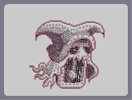Hover over the thumbnail for a full-size version.

Author SilentDemon author:silentdemon bitesized n-art rated 2006-06-10 4 by 51 people. \$Ghost#SilentDemon#none#90000000000000000000006000000000000000000000000000000000000000000000000000000000000000000000000000000000000000000000000000000000000000000000000000000000000000000000000000000000000000000000000000000000000000000000000000000000000000000000000000000000000000000000000000000000000000000000000000000000000000000000000000000000000000000000000000000000000000000000000000000000000000000000000000000000000000000000000000000000000000000000000000000000000000000000000000000000000000000000000000000000000000000000000000000000000000000000000000000000000000000000000000000000000000000000000000000000000000000000000000000000000000000000000000000000000000000000000000000000000000000000000000000000000000000080000000000000000000007|5^450,482!5^516,290!12^432,528!12^438,528!12^426,528!12^420,528!12^414,528!12^408,528!12^402,528!12^396,528!12^390,528!12^384,528!12^378,528!12^372,528!12^444,528!12^450,528!12^456,528!12^462,528!12^468,528!12^474,528!12^480,528!12^366,522!12^360,522!12^486,522!12^492,522!12^498,516!12^504,510!12^504,504!12^510,498!12^510,492!12^510,486!12^510,480!12^510,474!12^510,468!12^354,516!12^348,510!12^342,504!12^342,498!12^336,492!12^336,486!12^336,480!12^336,474!12^336,468!12^336,462!12^336,456!12^336,450!12^336,444!12^330,444!12^330,450!12^342,450!12^348,450!12^354,450!12^360,450!12^366,444!12^372,438!12^372,432!12^366,426!12^366,420!12^366,414!12^366,408!12^324,450!12^324,456!12^318,456!12^318,462!12^318,468!12^318,474!12^318,480!12^318,486!12^324,492!12^324,498!12^324,504!12^318,510!12^312,516!12^306,510!12^306,522!12^306,516!12^306,528!12^312,504!12^312,498!12^306,492!12^300,486!12^294,492!12^288,498!12^288,504!12^288,510!12^282,516!12^276,516!12^270,516!12^264,522!12^258,516!12^258,510!12^264,504!12^264,498!12^264,492!12^270,486!12^276,480!12^282,474!12^288,468!12^282,462!12^276,462!12^270,468!12^264,468!12^258,474!12^252,474!12^246,474!12^240,474!12^234,468!12^228,462!12^222,462!12^216,462!12^210,462!12^210,456!12^210,468!12^210,474!12^216,450!12^222,444!12^228,444!12^234,444!12^240,450!12^246,450!12^252,450!12^258,444!12^264,438!12^264,432!12^264,426!12^258,420!12^252,420!12^246,420!12^240,426!12^234,432!12^228,432!12^222,432!12^216,432!12^210,432!12^204,426!12^198,420!12^198,414!12^198,408!12^192,402!12^192,396!12^198,396!12^180,396!12^186,396!12^180,402!12^204,402!12^210,408!12^216,414!12^222,414!12^228,408!12^234,402!12^234,396!12^234,390!12^240,384!12^246,378!12^252,378!12^258,378!12^264,378!12^270,384!12^276,390!12^276,396!12^282,396!12^288,390!12^288,384!12^288,378!12^294,372!12^300,366!12^306,360!12^306,354!12^312,360!12^318,366!12^324,366!12^330,366!12^372,402!12^378,396!12^384,396!12^516,462!12^516,456!12^516,450!12^516,444!12^516,438!12^516,432!12^516,426!12^516,420!12^516,414!12^516,408!12^516,402!12^522,468!12^528,468!12^534,468!12^534,474!12^534,486!12^534,480!12^534,492!12^540,480!12^546,474!12^552,468!12^558,468!12^564,462!12^570,456!12^576,456!12^582,450!12^582,444!12^588,438!12^588,432!12^588,426!12^588,420!12^588,414!12^582,408!12^582,402!12^576,396!12^570,390!12^564,384!12^558,378!12^552,372!12^552,366!12^390,396!12^390,390!12^390,384!12^396,378!12^402,366!12^402,372!12^402,360!12^408,354!12^414,348!12^414,354!12^420,360!12^426,354!12^432,354!12^438,354!12^432,348!12^438,360!12^444,366!12^450,360!12^450,354!12^456,354!12^456,348!12^456,342!12^462,354!12^462,348!12^468,360!12^474,366!12^480,360!12^486,360!12^492,354!12^492,348!12^498,354!12^498,360!12^504,366!12^510,360!12^516,354!12^516,360!12^546,354!12^546,360!12^546,348!12^546,342!12^546,336!12^546,330!12^546,324!12^546,318!12^546,312!12^552,306!12^558,300!12^564,294!12^570,288!12^570,282!12^570,276!12^570,270!12^570,264!12^570,258!12^570,252!12^570,246!12^300,348!12^300,342!12^336,372!12^342,372!12^354,372!12^348,372!12^360,366!12^366,366!12^504,372!12^504,378!12^504,384!12^504,390!12^504,396!12^498,402!12^498,408!12^498,414!12^498,420!12^498,426!12^498,432!12^498,438!12^498,444!12^498,450!12^498,456!12^492,462!12^492,468!12^492,474!12^492,480!12^486,486!12^480,492!12^474,492!12^468,492!12^462,492!12^456,492!12^450,492!12^444,492!12^438,492!12^432,492!12^426,492!12^420,492!12^414,492!12^408,492!12^402,492!12^396,492!12^390,492!12^384,486!12^384,480!12^384,474!12^384,468!12^384,462!12^384,456!12^390,450!12^390,438!12^390,444!12^390,432!12^390,426!12^390,420!12^390,414!12^390,408!12^390,402!12^522,396!12^522,390!12^522,384!12^522,378!12^522,372!12^522,366!12^294,336!12^294,330!12^294,324!12^294,318!12^372,360!12^378,354!12^384,348!12^390,342!12^396,336!12^402,330!12^402,324!12^408,318!12^408,312!12^414,306!12^438,372!12^438,378!12^438,384!12^438,390!12^456,390!12^456,384!12^456,378!12^456,372!12^456,366!12^456,360!12^450,396!12^450,402!12^450,408!12^450,414!12^432,396!12^432,402!12^432,408!12^432,414!12^444,420!12^438,420!12^426,420!12^432,420!12^420,420!12^414,420!12^444,426!12^450,432!12^408,426!12^402,432!12^396,438!12^396,444!12^396,450!12^396,456!12^396,462!12^402,468!12^408,474!12^414,480!12^420,480!12^426,480!12^432,480!12^438,480!12^444,474!12^450,468!12^456,462!12^456,456!12^456,450!12^456,444!12^456,438!12^408,486!12^426,486!12^288,312!12^288,306!12^282,300!12^282,294!12^282,288!12^288,282!12^294,276!12^300,270!12^306,270!12^312,270!12^318,270!12^540,312!12^540,306!12^540,300!12^540,294!12^540,288!12^540,282!12^540,276!12^420,312!12^420,300!12^420,306!12^420,294!12^426,288!12^432,282!12^432,276!12^438,270!12^438,264!12^444,258!12^450,252!12^456,246!12^462,246!12^474,240!12^468,240!12^480,240!12^486,234!12^492,234!12^498,234!12^504,234!12^510,234!12^516,234!12^522,234!12^528,240!12^534,240!12^534,246!12^534,252!12^534,258!12^534,264!12^534,270!12^540,252!12^546,264!12^564,240!12^564,234!12^564,228!12^558,222!12^552,216!12^552,210!12^546,210!12^540,210!12^540,204!12^324,270!12^318,276!12^318,264!12^318,258!12^318,252!12^312,282!12^306,282!12^312,246!12^312,240!12^306,234!12^300,228!12^294,222!12^288,216!12^282,NaN!12^282,210!12^276,204!12^270,204!12^264,204!12^252,204!12^258,204!12^246,204!12^426,318!12^432,318!12^438,318!12^444,318!12^450,318!12^456,312!12^462,306!12^462,300!12^468,294!12^468,288!12^474,282!12^468,282!12^462,282!12^456,282!12^450,282!12^462,294!12^456,294!12^450,294!12^444,294!0^450,300!12^438,294!12^438,300!12^438,306!12^438,312!12^444,306!12^450,306!12^456,306!12^450,312!12^444,312!12^432,312!12^426,312!12^426,306!12^432,306!12^426,300!12^432,300!12^432,294!12^426,294!12^432,288!12^438,288!12^444,288!12^450,288!12^456,288!12^462,288!12^504,282!12^510,282!12^516,282!12^522,282!12^510,288!12^510,294!12^516,300!12^516,306!12^522,312!12^528,318!12^534,318!12^528,288!12^534,288!12^534,294!12^528,294!12^522,294!12^516,294!12^516,288!12^522,288!12^522,300!12^522,306!12^528,306!12^528,300!12^534,300!12^534,306!12^534,312!12^528,312!12^240,210!12^234,210!12^228,216!12^222,216!12^222,222!12^216,216!12^216,222!12^216,234!12^216,228!12^216,240!12^216,210!12^216,204!12^216,198!12^222,192!12^222,186!12^228,180!12^228,174!12^234,168!12^240,162!12^246,156!12^252,150!12^258,144!12^264,144!12^270,138!12^276,138!12^282,132!12^288,132!12^294,132!12^300,126!12^306,126!12^312,126!12^318,126!12^324,126!12^330,126!12^336,126!12^342,126!12^348,126!12^354,126!12^360,126!12^366,126!12^372,132!12^378,132!12^384,138!12^390,138!12^396,138!12^402,144!12^408,144!12^414,144!12^420,144!12^426,150!12^432,150!12^438,156!12^444,156!12^450,156!12^456,156!12^462,150!12^468,150!12^474,150!12^480,144!12^486,144!12^492,144!12^498,138!12^504,138!12^510,138!12^516,132!12^522,132!12^528,132!12^534,132!12^540,132!12^546,132!12^552,132!12^558,132!12^564,132!12^570,132!12^576,138!12^582,138!12^588,138!12^594,144!12^600,144!12^606,150!12^612,156!12^612,162!12^618,168!12^618,174!12^624,180!12^624,186!12^624,192!12^624,198!12^624,210!12^624,204!12^624,216!12^624,222!12^618,204!12^618,198!12^612,198!12^558,204!12^564,198!12^606,192!12^600,192!12^594,192!12^588,192!12^582,192!12^576,192!12^570,192!12^534,198!12^528,192!12^528,186!12^522,180!5^462,288!5^456,288!5^458,289!5^432,294!5^434,294!5^426,297!5^426,299!5^426,300!5^426,303!5^426,310!5^426,307!5^426,305!5^426,311!5^428,311!5^431,311!5^433,314!5^435,313!5^437,313!5^439,313!5^442,313!5^443,313!5^443,313!5^445,313!5^514,289!5^516,289!5^516,289!5^518,289!5^519,289!5^519,295!5^521,296!5^524,296!5^527,296!5^529,296!5^531,296!5^532,296!5^535,296!5^532,295!5^530,295!5^527,295!5^521,303!5^523,302!5^527,305!5^527,309!5^533,309!5^535,309!5^529,308!5^533,295!5^533,293!5^533,296!5^533,299!5^522,295!5^518,293!5^514,291!5^515,293!5^515,293!5^515,291!5^521,290!5^521,290!10^455,309!10^452,311!10^446,311!3^425,471!3^417,468!3^408,462!3^408,452!3^410,441!3^418,447!3^421,458!3^433,465!3^440,469!3^439,452!3^429,439!3^420,431!3^406,455!3^409,459!3^412,468!3^415,469!3^417,476!3^429,477!3^420,477!3^412,470!3^403,456!3^400,454!3^435,408!3^437,400!3^444,388!3^444,381!3^443,372!3^414,486!3^404,448!3^407,433!3^414,431!3^410,433!3^404,439!3^404,441!3^404,447!3^404,467!3^416,478!3^530,387!3^532,390!3^536,391!3^538,395!3^542,395!3^544,396!3^545,401!3^547,401!3^549,402!3^550,407!3^552,407!3^554,407!3^554,407!3^556,407!3^556,407!3^558,411!3^559,414!3^561,416!3^561,417!3^561,422!3^561,425!3^561,431!3^560,432!3^559,435!3^559,440!3^555,441!3^553,445!3^551,448!3^547,449!3^545,451!3^543,454!3^540,456!3^538,459!3^535,459!3^533,459!3^530,459!3^529,459!3^528,459!3^526,459!3^526,458!3^526,454!3^526,451!3^526,451!3^526,449!3^526,448!3^526,446!3^526,445!3^526,443!3^526,442!3^526,442!3^526,440!3^526,440!3^526,437!3^526,436!3^526,435!3^526,434!3^526,434!3^526,434!3^526,431!3^526,431!3^526,429!3^526,428!3^526,427!3^526,422!3^526,422!3^526,421!3^526,419!3^526,417!3^526,417!3^526,415!3^526,413!3^526,409!3^526,408!3^526,406!3^526,406!3^529,406!3^529,403!3^529,400!3^533,400!3^533,398!3^539,409!3^539,409!3^536,410!3^536,410!3^536,412!3^536,415!3^536,415!3^536,416!3^536,418!3^536,420!3^536,420!3^536,422!3^536,423!3^536,424!3^536,426!3^536,426!3^536,427!3^535,427!3^535,430!3^535,433!3^535,434!3^535,435!3^535,438!3^535,442!3^535,445!3^536,447!3^536,448!3^542,443!3^544,440!3^545,440!3^545,439!3^545,438!3^546,437!3^547,437!3^547,435!3^547,434!3^547,432!3^547,432!3^547,431!3^547,428!3^547,426!3^547,424!3^547,423!3^547,421!3^547,419!3^547,414!3^546,414!3^545,412!3^545,411!3^543,407!3^532,393!3^531,390!3^529,387!3^556,417!3^557,424!3^556,425!3^553,428!3^557,432!3^554,439!3^549,445!3^545,447!3^539,448!3^344,456!3^344,459!3^344,462!3^344,464!3^344,466!3^344,468!3^344,471!3^344,474!3^344,476!3^344,479!3^344,480!3^344,482!3^344,483!3^344,485!3^344,488!3^344,490!3^358,460!3^358,468!3^358,479!3^358,489!3^358,500!3^358,510!3^350,503!3^373,461!3^373,483!3^376,505!3^381,524!3^382,442!3^377,421!3^382,402!3^203,421!3^209,425!3^221,428!3^232,425!3^241,414!3^254,409!3^235,419!3^247,405!3^250,395!3^258,396!3^263,405!3^260,413!3^273,407!3^269,416!3^313,371!3^308,380!3^303,388!3^304,397!3^295,407!3^282,411!3^325,378!3^317,388!3^317,398!3^313,408!3^306,413!3^297,420!3^285,420!3^274,428!3^280,420!3^222,455!3^231,455!3^239,459!3^247,460!3^258,454!3^263,446!3^273,441!3^280,439!3^286,427!3^297,431!3^286,439!3^289,444!3^300,444!3^268,494!3^275,490!3^281,482!3^268,500!3^288,475!3^293,452!3^296,451!3^298,458!3^300,467!3^306,436!3^337,388!3^335,406!3^327,423!3^348,434!3^348,417!3^352,401!3^363,437!3^311,424!3^353,378!3^573,431!3^570,442!3^564,452!3^554,461!3^542,467!3^395,525!3^409,524!3^425,524!3^441,524!3^456,524!3^474,524!3^518,243!3^512,243!3^505,243!3^498,243!3^492,243!3^483,243!3^473,245!3^464,249!3^457,254!3^469,254!3^480,254!3^489,254!3^498,254!3^501,254!3^511,253!3^518,253!3^526,249!3^526,259!3^516,243!3^509,243!3^507,243!3^503,243!3^496,243!3^491,243!3^487,243!3^510,244!3^519,244!3^520,244!3^515,265!3^505,265!3^496,264!3^485,265!3^475,265!3^466,265!3^454,265!3^446,268!3^442,279!3^453,274!3^465,275!3^475,277!3^488,277!3^501,276!3^516,276!3^528,270!3^531,280!3^477,251!3^466,254!3^457,257!3^477,251!3^495,251!3^517,254!3^498,254!3^484,254!3^477,253!3^504,250!3^516,250!3^514,243!3^507,243!3^501,243!3^495,243!3^493,243!3^489,243!3^486,242!5^490,241!5^504,241!5^517,241!3^498,289!3^507,298!3^514,312!3^525,317!3^514,305!3^525,321!3^536,322!3^502,283!3^477,287!3^471,296!3^467,307!3^462,316!3^454,326!3^442,326!3^426,324!3^417,315!3^493,340!3^499,348!3^413,326!3^403,337!3^393,346!3^385,351!3^324,444!3^377,362!3^535,385!3^545,391!3^553,397!3^560,405!3^564,415!3^524,354!3^521,339!3^528,330!3^535,342!3^535,366!3^508,337!3^441,344!3^421,347!3^473,351!3^476,313!3^471,334!3^242,202!3^254,197!3^264,197!3^279,202!3^293,212!3^308,225!3^317,244!3^325,261!3^321,277!3^307,290!3^306,280!3^309,277!3^302,280!3^302,280!3^301,281!3^299,282!3^298,285!3^298,287!3^295,287!3^293,287!3^291,290!3^291,293!3^293,295!3^294,295!3^294,298!3^294,299!3^294,301!3^296,306!3^299,312!3^301,315!3^302,317!3^303,320!3^303,322!3^303,326!3^303,330!3^304,332!3^305,339!3^307,342!3^309,347!3^311,350!3^314,354!3^315,356!3^317,357!3^317,357!3^322,359!3^323,359!3^397,505!3^421,505!3^444,505!3^466,505!3^493,500!3^494,482!3^497,463!3^502,448!3^501,425!3^502,406!3^507,382!3^314,340!3^323,340!3^329,339!3^337,339!3^344,338!3^349,334!3^353,332!3^354,327!3^355,325!3^352,335!3^349,335!3^344,337!3^339,337!3^332,337!3^325,337!3^319,337!3^314,334!3^313,331!3^313,322!3^313,322!3^313,319!3^309,311!3^308,303!3^308,298!3^305,293!3^305,293!3^303,291!3^302,291!3^304,296!3^305,301!3^308,303!3^314,307!3^320,310!3^324,311!3^318,318!3^321,318!3^314,318!3^316,323!3^315,325!3^319,325!3^323,322!3^331,321!3^334,332!3^333,325!3^342,325!3^339,323!3^334,319!3^333,312!3^330,310!3^323,304!3^318,297!3^316,292!3^316,288!3^315,283!3^315,283!3^325,299!3^333,305!3^329,325!3^329,329!3^323,329!3^314,323!3^309,315!3^305,305!3^300,304!3^292,300!3^286,293!3^289,289!3^292,285!3^300,281!3^303,276!3^313,276!3^313,283!3^319,290!3^319,298!3^322,303!3^323,303!3^326,303!3^331,304!3^331,309!3^332,313!3^335,316!3^352,327!3^352,327!3^352,327!3^348,322!3^344,317!3^340,314!3^314,328!3^310,332!3^310,345!3^313,350!3^319,353!3^328,351!3^331,359!3^336,348!3^339,359!3^344,366!3^357,365!3^337,368!3^351,357!3^347,348!3^356,348!3^364,356!3^366,343!3^357,339!3^373,355!3^374,345!3^369,332!3^381,335!3^384,343!3^391,331!3^377,323!3^390,318!3^367,323!3^360,313!3^359,320!3^364,309!3^366,305!3^367,301!3^367,299!3^367,295!3^367,295!3^368,287!3^370,285!3^371,283!3^375,279!3^377,279!3^374,283!3^371,283!3^369,285!3^368,285!3^367,286!3^364,291!3^361,294!3^360,296!3^359,297!3^358,298!3^373,288!3^372,291!3^371,292!3^367,294!3^357,298!3^350,300!3^348,301!3^348,305!3^349,306!3^350,306!3^351,307!3^356,308!3^359,307!3^356,307!3^346,307!3^342,307!3^342,307!3^342,302!3^342,299!3^339,297!3^336,296!3^378,200!3^373,204!3^367,205!3^363,209!3^363,211!3^363,216!3^363,218!3^364,225!3^365,228!3^373,234!3^375,237!3^376,244!3^376,252!3^376,259!3^376,271!3^383,271!3^384,267!3^388,262!3^395,257!3^402,252!3^404,250!3^408,248!3^409,242!3^414,239!3^363,207!3^354,207!3^349,207!3^343,207!3^337,205!3^336,205!3^333,203!3^328,201!3^326,201!3^320,199!3^315,196!3^307,195!3^305,193!3^298,193!3^296,193!3^290,193!3^281,193!3^275,193!3^267,193!3^260,193!3^250,196!3^240,197!3^235,201!3^235,204!3^229,205!3^228,207!3^228,208!3^226,209!3^224,210!3^246,193!3^262,192!3^277,192!3^289,193!3^298,193!3^310,193!3^362,234!3^367,239!3^372,242!3^373,243!3^373,245!3^375,247!3^375,250!3^375,258!3^375,262!3^374,264!3^374,267!3^376,271!3^397,255!3^393,257!3^385,257!3^384,259!3^382,263!3^380,265!3^377,269!3^376,273!3^365,230!3^363,230!3^363,230!3^361,229!3^361,223!3^361,219!3^355,213!3^354,210!3^353,208!3^344,208!3^338,208!3^288,202!3^281,197!3^285,202!3^289,205!3^295,208!3^299,208!3^302,208!3^298,209!3^308,213!3^300,214!3^305,217!3^305,223!3^305,223!3^311,224!3^314,227!3^315,228!3^315,233!3^319,235!3^320,237!3^322,239!3^322,240!3^322,246!3^323,250!3^324,252!3^324,261!3^324,270!3^324,275!3^324,278!3^324,281!3^324,283!3^301,223!3^301,223!3^291,211!3^284,203!3^270,196!3^272,196!3^282,200!3^292,200!3^307,204!3^310,206!3^312,206!3^317,206!3^318,206!3^311,205!3^300,199!3^300,199!3^306,199!3^310,199!3^327,206!3^329,211!3^332,212!3^332,214!3^340,214!3^344,215!3^347,217!3^350,217!3^352,218!3^354,218!3^358,218!3^360,219!3^360,226!3^360,234!3^360,248!3^360,253!3^362,253!3^364,253!3^366,253!3^366,253!3^367,253!3^368,253!3^368,253!3^368,262!3^368,286!3^361,274!3^366,274!3^367,274!3^367,271!3^367,262!3^367,259!3^364,259!3^363,252!3^364,245!3^361,240!3^359,239!3^358,237!3^356,236!3^352,234!3^347,222!3^343,219!3^341,219!3^334,219!3^328,219!3^322,219!3^317,217!3^316,215!3^315,215!3^313,215!3^317,213!3^319,223!3^327,224!3^330,229!3^332,232!3^333,237!3^333,248!3^331,250!3^332,259!3^332,273!3^332,276!3^332,280!3^332,282!3^332,284!3^330,284!3^330,284!3^330,285!3^330,286!3^330,287!3^330,288!3^341,284!3^355,284!3^356,284!3^356,276!3^351,268!3^346,265!3^338,273!3^333,262!3^333,254!3^336,243!3^341,233!3^349,235!3^349,243!3^343,255!3^338,257!3^337,260!3^336,265!3^328,254!3^330,249!3^340,230!3^348,227!3^346,231!3^338,228!3^339,233!3^344,251!3^348,256!3^356,260!3^350,245!3^342,240!3^346,255!3^354,252!3^350,254!3^351,262!3^351,267!3^349,269!3^353,279!3^353,280!3^347,282!3^342,283!3^322,259!3^327,259!3^346,301!3^348,290!3^353,290!3^353,283!3^355,270!3^363,266!3^364,272!3^362,275!3^359,268!3^355,258!3^336,295!3^337,269!3^342,245!3^341,240!3^329,228!3^322,220!3^319,219!3^317,218!3^534,182!3^540,182!3^543,183!3^549,183!3^558,183!3^564,183!3^571,183!3^578,188!3^563,189!3^559,193!3^555,184!3^549,184!3^545,186!3^538,186!3^537,186!3^539,190!3^542,193!3^543,195!3^547,198!3^549,199!3^551,202!3^551,204!3^554,198!3^554,193!3^555,190!3^377,307!3^335,349!3^325,353!3^344,363!3^336,356!3^353,357!3^401,310!3^391,304!3^384,294!3^390,280!3^399,292!3^407,302!3^412,288!3^405,279!3^397,262!3^592,187!3^583,182!3^580,176!3^572,171!3^564,170!3^559,170!3^544,171!3^537,175!3^551,171!3^556,171!3^567,174!3^573,182!3^541,263!3^545,272!3^547,277!3^548,281!3^550,287!3^551,289!3^551,293!3^552,296!3^552,297!3^552,299!3^548,305!3^546,267!3^548,276!3^389,193!3^405,184!3^228,192!3^240,180!3^260,179!3^281,179!3^296,178!3^270,181!3^250,182!3^236,191!3^308,182!3^288,183!3^321,188!3^334,193!3^347,195!3^363,195!3^358,185!3^346,182!3^333,179!3^318,171!3^303,165!3^285,166!3^271,168!3^255,167!3^242,169!3^327,163!3^341,168!3^352,165!3^361,174!3^314,167!3^372,184!3^383,184!3^377,170!3^265,153!3^284,152!3^300,150!3^319,148!3^339,150!3^357,148!3^374,157!3^390,166!3^406,173!3^378,202!3^388,196!3^403,185!3^397,191!3^409,198!3^400,203!3^392,207!3^379,214!3^379,226!3^389,243!3^387,234!3^398,237!3^409,228!3^394,226!3^396,214!3^385,220!3^405,219!3^411,208!3^408,268!3^426,269!3^419,278!3^396,269!3^420,263!3^430,258!3^440,250!3^451,243!3^418,214!3^421,227!3^431,220!3^431,208!3^439,212!3^451,207!3^426,195!3^378,216!3^381,225!3^388,238!3^396,241!3^415,187!3^418,203!3^442,202!3^429,178!3^444,179!3^461,178!3^479,168!3^501,163!3^521,157!3^541,157!3^563,158!3^580,169!3^599,177!3^426,244!3^458,234!3^443,232!3^468,219!3^495,216!3^534,215!3^559,238!3^567,264!3^475,231!3^490,228!3^505,228!3^519,230!3^531,235!3^541,244!3^548,257!3^453,197!3^433,201!3^463,207!3^476,203!3^488,203!3^500,205!3^512,206!3^524,206!3^464,194!3^474,191!3^487,191!3^501,192!3^515,181!3^512,193!3^521,194!3^500,184!3^575,160!3^593,171!5^493,369!5^493,371!5^493,373!5^493,374!5^492,376!5^491,379!5^489,372!5^486,372!5^485,372!5^483,372!5^481,378!5^476,378!5^476,378!5^471,377!5^466,376!5^466,373!5^464,368!5^469,374!5^466,375!5^464,380!5^465,388!5^471,388!5^475,388!5^480,387!5^484,387!5^488,387!5^494,387!5^496,387!5^498,387!5^498,387!5^492,387!5^480,387!5^476,386!5^472,384!5^466,390!5^458,400!5^461,400!5^464,400!5^464,400!5^464,400!5^467,400!5^469,401!5^475,402!5^475,402!5^475,402!5^479,402!5^480,402!5^482,402!5^484,402!5^486,402!5^489,401!5^488,398!5^488,398!5^488,397!5^459,407!5^459,409!5^460,411!5^460,417!5^460,420!5^460,420!5^460,424!5^460,425!5^455,425!5^458,425!5^460,425!5^461,425!5^463,425!5^468,425!5^470,421!5^470,416!5^470,414!5^470,414!5^475,416!5^482,416!5^485,418!5^486,414!5^489,411!5^489,420!5^488,428!5^488,431!5^486,435!5^486,441!5^486,443!5^486,443!5^486,446!5^486,449!5^486,450!5^486,453!5^479,477!5^480,476!5^481,474!5^481,472!5^481,472!5^481,469!5^481,466!5^481,462!5^481,462!5^478,456!5^477,454!5^476,447!5^472,442!5^472,440!5^472,438!5^472,438!5^472,435!5^472,432!5^472,429!5^466,435!5^466,440!5^466,444!5^466,446!5^466,449!5^466,451!5^466,453!5^466,455!5^466,457!5^466,459!5^466,461!5^466,464!5^466,466!5^466,466!5^466,470!5^465,470!5^465,472!5^465,476!5^465,477!5^465,477!5^465,477!5^465,477!5^465,480!5^465,482!5^469,482!5^475,482!5^475,482!5^477,482!5^478,482!5^458,473!5^457,474!5^453,481!5^452,483!5^455,484!5^460,483!5^461,482!5^463,482!5^464,482!5^406,482!5^401,482!5^400,479!5^398,474!5^392,464!5^392,468!5^392,469!5^392,472!5^392,477!5^393,482!5^400,420!5^400,417!5^400,416!5^400,410!5^402,406!5^402,403!5^402,399!5^399,386!5^396,419!5^397,389!5^401,391!5^403,391!5^406,391!5^407,387!5^408,385!5^409,381!5^409,379!5^410,372!5^411,368!5^411,364!5^411,362!5^414,364!5^429,365!5^432,365!5^433,365!5^434,365!5^429,366!5^427,369!5^426,372!5^425,374!5^423,374!5^423,375!5^421,375!5^418,374!5^413,366!5^404,404!5^407,404!5^408,408!5^409,408!5^411,408!5^412,408!5^412,408!5^413,408!5^414,408!5^416,408!5^416,408!5^417,408!5^419,408!5^420,408!5^420,408!5^421,408!5^422,408!5^422,408!5^422,408!5^424,408!5^425,408!5^425,408!5^425,406!5^425,403!5^425,399!5^425,397!5^425,395!5^425,393!5^425,390!5^425,388!5^424,387!5^424,387!5^432,387!5^432,387!5^428,387!5^421,388!5^416,388!5^415,388!5^411,390!5^400,482!5^433,487!5^438,487!5^439,487!5^439,487!5^440,487!5^441,487!5^444,487!5^444,487!5^446,487!5^448,487!5^451,483# -repost- last request from nemetacyst

## Other maps by this author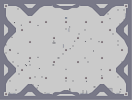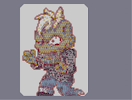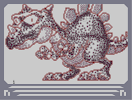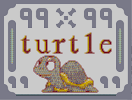Can't last forever Zombie Drake 99turtle99

Pages: (0)

i see you.

### i see you.

i see you.
yea I'm shook that its been that long

### Time does indeed fly...

Nine years since my last comment here.

### ...oh wait

You commented here a few days ago too.
Hm.

### ...

I think it's really funny Ska looked you up just two days before I did.
I love you; if you ever come back be sure to comment at me or something. I'm not around often, but anyway. <3

### hey

thanks for the shout out on your profile. It's not often I get name-checked at all, so thanks and good luck with life!
Cheers :)
Sup dude, the new forums are right here:
http://forum.droni.es/

### Oh...

I just remember seeing you a long time ago.

(I joined in '08, but saw a bunch of old maps like this.)

TEH CHRONOS~

Nice.

5/5 man

U play ms a lot?

### Ahh I love it!

Mmm... a Platoon Chronos...

Is that right? yeah, I think so...
Anyways, 5aved for awesomeness.

### maplestory...

thats a monster from maple story but 5/5

### Jig,

that was quite uncharacteristic...

### I love it

I really like it. I like the whole concept of the picture, the shading and all. Well done, 5aved =)

### jig

wtf does that mean?

### thanks demon

i got all of them...its a shame to delete these(again)...but do whatever you feel is best, or what you want...
you were a hell of an artist, good bye and good luck...

### umm

no cuz nemetacyst wants to see it, and it'll me delte soon and i don't think i got sniped that much. i don't really care about the ratings anyway.

### Let me guess...

You resubmitted it because it got "sniped"?
Because frankly, I've grown ridiculously tired of this style of N-art. And I think this deserves a 3.5 at maximum. You need to branch out and make maps other than droll N-arts in the same style each time. This category shows absolutely no skill whatsoever, and if you can't make real maps, you're wasting the time of the entire community.

### Once again..

5aved. Amazing work - this being my favorite of them all.

### dc

was that really necessary?

### Holy fuck dude

how many of these do you have?

### 5/5

Man. Your amazing. All of this n art. Its amazing. 5/5. Without doubt.